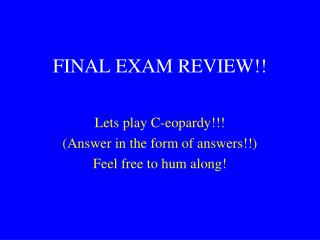DownloadDownload PresentationFINAL EXAM REVIEW!!

# FINAL EXAM REVIEW!!

Download Presentation## FINAL EXAM REVIEW!!

- - - - - - - - - - - - - - - - - - - - - - - - - - - E N D - - - - - - - - - - - - - - - - - - - - - - - - - - -
##### Presentation Transcript

1. FINAL EXAM REVIEW!! Lets play C-eopardy!!! (Answer in the form of answers!!) Feel free to hum along!

2. Arrays Functions & Macros Strings Pointers Loops 100 100 100 100 100 200 200 200 200 200 300 300 300 300 300

3. Question: Given the following: function prototype: void my_func(int array[]); variable declaration: int X; Which function call can you use to pass a 2-dimensional array to a function? my_func(X); my_func(&X); my_func(X);

5. Question What is printed: int a={0}, i,j; for(i=0;i<3;i++) for(j=i;j<3;j++) a[i][j]=i*j; for(i=0;i<3;i++) printf(“%d\t”,a[i]);

7. Question What is printed: int a={0}, n=27212, abc; while(n>0) { abc=n%10; if(a[abc]) { printf(“%d\t”,abc); break; } a[abc]=1; n/=10; }

8. Question Given the following: #define DOUBLE(x) (2*x) What is the output for printf(“%d”,DOUBLE(1+2));

9. Question Write the following equation as a C statement using functions from the math.h library. y=(en ln(b))2

11. Question Given the following program: main() { int n = 10; printf(“%d”,funct1(n)); } int funct1(int n) { if(n>0) return(n + funct1(n-1)); } What is the o/p ?

13. Question Given the following program: #include <stdio.h> #define ROWS 3 #define COLUMNS 4 int z[ROWS][COLUMNS] = {1,2,3,4,5,6,7,8,9,10,11,12}; int main() { int a, b, c; for(a=0;a<ROWS,++a) { c = 999; for(b=0;b<COLUMNS;++b) if(z[a][b] < c) c = z[a][b]; printf(“%d ”,c); } What is the o/p?

14. Answer 1 5 9 (smallest value within each row)

15. Question What is the output of the following code fragment: inti; char x = “gorilla”; char y = “giraffe”; char z; strcpy(z,y); strcpy(z,strcat(x,y)); strcpy(z,x); for(i=0;i<3;i++) printf(“%s\n”,z[i]);

17. Question What is the final value of string s1? strcpy(s1,"computer"); strcpy(s2,"science"); if(strcmp(s1,s2) < 0) strcat(s1,s2); else strcat(s2,s1); s1[strlen(s1)-6] = '\0';

19. Question If ‘i’ is a variable and ‘p’ points to ‘i’, which of the following expression are aliases for ‘i’ ? *p e) *i &p f) &i *&p g) *&i &*p h) &*i

21. Question Consider following declaration: float table = { {1.1, 1.2, 1.3}, {2.1, 2.2, 2.3} }; What is the value of ? *(*(table+1));

23. Question Given: int x[]={-1,-2,-3,-4}, *px, **ppx; px=x; ppx=&px; Which command will display -3. A: printf(“%d”,**ppx+2); B: printf(“%d”,*(*ppx+2)); C: printf(“%d”,*ppx+2); D: printf(“%d”,*(ppx+2));

24. Question Given the following: int i=0,n=465; do { n/=10; i++; } while(n>0); printf(“i=%d\n”,i); What is printed?

25. Question What is printed: int i=10; while(i-- +2); printf(“%d”,i);